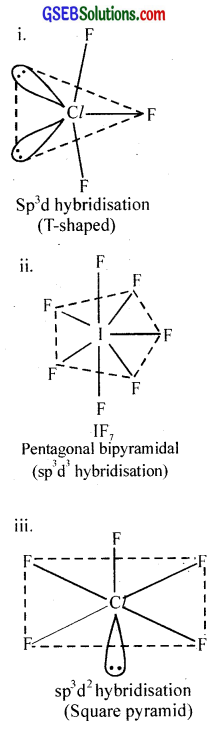# GSEB Solutions Class 12 Chemistry Chapter 7 The p-Block Elements

Gujarat Board GSEB Textbook Solutions Class 12 Chemistry Chapter 7 The p-Block Elements Textbook Questions and Answers, Additional Important Questions, Notes Pdf.

## Gujarat Board Textbook Solutions Class 12 Chemistry Chapter 7 The p-Block Elements

### GSEB Class 12 Chemistry The p-Block Elements InText Questions and Answers

Question 1.
Why are pentahalides more covalent than trihalides?
Higher the positive oxidation state of the central atom, it will have more polarising power which, in turn, will increase the covalent character of the bond formed between the central atom and the other atom. Hence pentahalides are more covalent than trihalides.Question 2.
Why is BiH3 the strongest reducing agent amongst all the hydrides of Group 15 elements?
On moving down the group, the E – H bond weakens and hence reducing property increases. BiH3 is the least stable among the hydrides and is the strongest reducing agent.

Question 3.
Why is N2 less reactive at room temperature?
In N2 molecule, there is a strong pπ-pπ overlap that results in the formation of triple bond between N atoms (N ≡ N). As a consequence, the bond dissociation energy of N2 is very high and N2 is less reactive at room temperature.

Question 4.
Mention the conditions required to maximise the yield of ammonia?

1. Low temperature: The optimum temperature is 650 – 800 K.
2. High pressure: The optimum pressure is 200 atm.
3. Catalyst: Finely divided iron with molybdenum as promotor.

Question 5.
How does ammonia react with a solution of Cu2+?
Ammonia reacts with Cu2+ to from a deep blue coloured complex.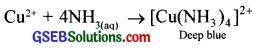Question 6.
What is the covalence of nitrogen in N2O5?
The structure of N2O5 is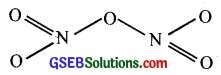From the structure of N2O5 it is evident that covalence of nitrogen is four.

Question 7.
Bond angle in PH+4 is higher than that in PH3. Why?
PH4+ is a regular tetrahedral structure, thus the bond angle is 109°28′.
PH3 molecule has a pyramidal structure. The bond angle is 93°. Hence, bond angle in PH4+ ion is higher.

Question 8.
What happens when white phosphorus is heated with concentrated NaOH solution in an inert atmosphere of CO2?
When white phosphorus is heated with concentrated NaOH solution in an inert atmosphere gives phosphine.
P4 + 3NaOH + 3H2O → PH3 + 3NaH2PO2

Question 9.
What happens when PCl5 is heated?
PCl5 on strong heating decomposes to give PCl3 and Cl2.
PCl5 → PCl3 + Cl2Question 10.
Write a balanced equation for the hydrolytic reaction of PCl5 in heavy water.
PCl5 + D2O → POCl3 + 2DCl

Question 11.
What is the basicity of H3PO4?
The structure of H3PO4 is image 3. Since there are 3 P – OH groups, the basicity of H3PO4 is three.

Question 12.
What happens when H3PO3 is heated?
H3PO3 disproportionates on heating to give orthophosphoric acid, H3PO4 and phosphine, PH3. 4H3PO3 → 3H3PO4 + PH3

Question 13.
List the important sources of sulphur?
Sulphates and sulphides are important sources of sulphur.
Sulphates: Gypsum (CaSO4.2H2O), Epsom salt (MgSO4.7H2O), Baryte (BaSO4).
Sulphides: Galena (PbS), Zinc blende (ZnS), Copper pyrites (CuFeS2), Iron pyrites (FeS2) etc.
Organic materials such as eggs, proteins, garlic, onion, mustard, hair and wool also contain sulphur in small quantities.

Question 14.
Write the order of thermal stability of the hydrides of Group 16 elements?
H2O > H2S > H2Se > H2Te > H2POQuestion 15.
Why is H2O a liquid and H2S a gas?
Due to high electronegativity of oxygen and its small size, there are strong H-bonding in water. As a result, the molecules exist as associated and is liquid at room temperature. But there is no H-bonding in H2S because of low electronegativity of ‘S’.

Question 16.
Which of the following does not react with oxygen directly?
Zn, Ti, Pt, Fe
Platinum (Pt)

Question 17.
Complete the following reactions:

1. C2H4 + O2
2. 4Al + 3O2

1. C2H4 + 3O2 → 2CO2 + 2H2O
2. 4Al + 3O2 → 2Al2O3

Question 18.
Why does O3 act as a powerful oxidising agent?
O3 decomposes to give nascent oxygen and hence acts as a powerful oxidising agent.
O3 → O2 + [O]Question 19.
How is O3 estimated quantitatively?
Ozone oxidises potassium iodide solution to iodine.
2KI + H2O + O3 → 2KOH + I2 + O2
When ozone is treated with excess of potassium iodide solution buffered with a borate buffer (pH = 9.2), iodine is liberated which can be titrated against a standard solution of sodium thiosulphate solution using starch as indicator. This is a quantitative method to estimate ozone.

Question 20.
What happens when sulphur dioxide is passed through an aqueous solution of Fe(III) salt?
Sulphur dioxide reduces Fe(III) ions to Fe(II) ions.
2Fe3+ + SO2 + 2H2O → 2Fe2+ + SO2-4 + 4H+

Question 21.
Comment on the nature of two S – O bonds formed in SO2 molecule. Are the two S – O bonds in this molecule equal?
Both the S-O bonds are covalent and have equal strength due to resonating structures.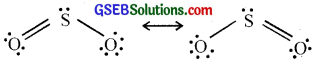Question 22.
How is the presence of SO2 detected?
Moist sulphur dioxide behaves as a reducing agent. SO2 decolourises acidified potassium permanganate solution.
5SO2 + 2MnO4 + 2H2O → 5SO2-4 + 4H+ + 2Mn2+

Question 23.
Mention three areas in which H2SO4 plays an important role?
Uses of H2SO4:

• Manufacture of fertilizers (e.g. ammonium sulphate, calcium superphosphate)
• Petroleum refining
• Metallurgical applications (e.g. cleansing metals before enamelling electroplating, galvanising etc.)

Question 24.
Write the conditions to maximise the yield of H2SO4 by contact process?

1. Low temperature as the conversion of SO2 to SO3 is exothermic (720K).
2. High pressure (2 atm).
3. A catalyst (Pt or V2O5) to increase the rate of reaction.Question 25.
Why is Ka2 << Ka1 for H2SO4 in water?
H2SO4 is a very strong acid in water largely because of its first ionisation to H3O+ and HSO4. The ionisation of HSO4 to H3O+ and SO4 is very very small. That is why Ka2 << Ka1.

Question 26.
Give two examples to show the anomalous behaviour of fluorine?
Two anomalous behaviour of fluorine are:
(i) Since fluorine is a most electronegative element, it shows only a negative oxidition state of-1. It does not show any positive oxidation state. On the other hand, the other halogens show positive oxidation states also such as +1, +3, +5, +6 and +7.
(ii) Maximum covalency of fluorine is one because it can not expand its valence shell beyond octet because there are no d-orbitals in the valence shell. On the other hand, other elements can exercise covalencies up to 7 because of the availability of vacant d-orbitals.

Question 27.
Sea is the greatest source of some halogens. Comment?
Seawater contains chlorides, bromides, and iodides of Na, K, Mg and Ca. Seawater contains NaCl at about 2.5% by mass. Seaweeds contain 0.5% of iodine.

Question 28.
Give the reason for the bleaching action of Cl2.
Bleaching action of chlorine is due to its oxidation. In the presence of moisture, chlorine gives nascent oxygen.
Cl2 + H2O → 2HCl + [O]
Because of nascent oxygen, it bleaches colouring substance as
Colouring substance + [O] → Colourless substance.
It bleaches vegetables or organic matter. The bleaching action of chlorine is permanent.

Question 29.
Name two poisonous gases which can be prepared from chlorine gas?

1. Phosgene (COCl2)
2. Tear gas (CCl3, NO2)

Question 30.
Why is ICl more reactive than If?
In general, interhalogen compounds are more reactive than halogens due to weaker X – X’ bonding than X – X bond. Thus, ICl is more reactive than I2.Question 31.
Why is helium used in the diving apparatus?
Helium is used as a diluent for oxygen in modern diving apparatus because of its very low solubility in blood.

Question 32.
Balance the following equation:
XeF6 + H2O → XeO2F2 + HF
XeF6 + 2H2O → XeO2F2 + 4HF

Question 33.
Why has it been difficult to study the chemistry of radon?
Radon is radioactive with a very short half-life of 3-82 day’s that’s why the study of the Chemistry of Radon is difficult.

### GSEB Class 12 Chemistry The p-Block Elements Text Book Questions and Answers

Question 1.
Discuss the general characteristics of Group 15 elements with reference to their electronic configuration, oxidation state, atomic size, ionisation enthalpy and electronegativity?
The group-15 of the periodic table contains five elements, namely nitrogen (N), phosphorus (P), arsenic (As), antimony (Sb) and bismuth (Bi). These elements are called ‘Pnicogens’ and their compounds as ‘Pniconides’. The name is derived from the Greek word ‘Pnicogens’ meaning suffocation.

Question 2.
Why does the reactivity of nitrogen differ from phosphorus?
Because of its small size, nitrogen can form π bonds and hence it exists as a diatomic molecule with a triple bond. Since the bond dissociation energy of N2 is large, it is inert and unreactive. But phosphorus exists as P4 molecule and the P – P bond is weaker than N ≡ N
∴ Phosphorus is more reactive than nitrogen.Question 3.
Discuss the trends in chemical reactivity of group 15 elements.
Nitrogen is extremely inert. The triple bond in N ≡ N (N2) molecule is extremely stable and requires large amount of dissociation energy. Phosphorus is more reactive than nitrogen and hence in nature it is not found in free state. The high reactivity of phosphorus is due to the presence of weak single P – P covalent bond among phosphorus atoms in P4 molecule.

(i) Oxides:
The elements of group-15 combine with oxygen to form oxides in which they show +3 and +5 oxidation states. Nitrogen forms a number of oxides with oxidation numbers varying from +1 to + 5. These are nitrous oxide (N2O), nitric oxide (NO), dinitrogen trioxide (N2O3) nitrogen tetroxide or nitrogen dioxide (NO2 or N2O4), and dinitrogen pentoxide (N2O5).

N2O and NO are neutral while N2O3, N2O4 and N2O5 are purely acidic. The oxides of phosphorus P4O6, P4O8 and P4O10 are purely acidic and those of arsenic and antimony are amphoteric while that of bismuth is basic.

The acidic character of trionides and pentonides decreases with increase in atomic numbers. Thus, the acidic character is in the order N2O3 > P2O3 > As2O3 and N2O5 > P2O5 > As2O3

Acidic character increases with increase in the positive oxidation number. Thus the acidic strength of oxides of nitrogen is in the order N2O < NO <N2O3 < N2O4 < N2O5

(ii) Hydrides:
All the elements of group 15 combine with hydrogen giving volatile hydrides of the type EH3. They are NH3 (Ammonia), PH3 (Phosphine), AsH3 (Arsine), SbH3 (Stibine) and BiH3 (Bismuthine).

On moving down the group. cóvalent character, basicity and thermal stability of these hydrides decrease while the reducing character increases. The boiling point of ammonia unexpectedly high due to molecular association caused by the presence of intermolecular by hydrogen bonding.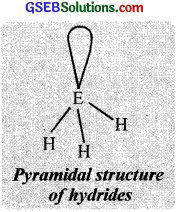Structure:
These hydrides have pyramidal structure in which central atom (sp3 hybridised state) holds a lone pair of electron in one of the of hydrides orbitais. The H-E-H angle is less than the original tetrahedral bond angle of 109°28’ because of greater repulsion between the lone pair and the bond pairs than between two bond pairs. Thus the H-N-H bond angle in NH3 is 107°.

As the electronegativity of the central atom decreases from N to Bi, the bond pairs lie farther away from the central atom, and the lone pair causes greater repulsion. Hence the H-P-H bond angle in PH3 is 93°, while the bond angle in AsH3 is 91° and SbH3 is 9o°.

(iii) Halides:
Except nitrogen, all the elements of this group form trihalides (EX3) and pentahalides (EX5). Nitrogen fonns triha lides only. Trihalides of nitrogen except NF3 are not stable. All trihalides except BiF3 are covalently bonded and they have pyramidal shape (sp3 hybridisation) in the gaseous state.

Nitrogen does not form pentahalides due to the absence of d-orbitals. In the gaseous state, the pentahalide (EX5) molecules possess trigonal bipyramidal structure due to sp3d hybridisation. The important pentahalides of this group are PCl5, PF5. SbF5 etc. Phosphorus pentaiodide does not exist because of the large size of iodine atoms and the small clectronegalivity difference between phosphorus and iodine.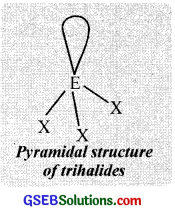Question 4.
Why does NH3 form hydrogen bond but PH3 does not?
The N – H bond in ammonia is quite polar as nitrogen is highly electronegative in nature. As a result, NH3 molecules are linked by intermolecular hydrogen bonding. On the other hand, P – H bond is non-polar as both P and H have the same electronegativity. Hence in phosphine, no hydrogen bonding is present.

Question 5.
How is nitrogen prepared in the laboratory? Write the chemical equations of the reactions involved?
Nitrogen was discovered by Daniel Rutherford in 1772.

Preparation:
(I) In the laboratory, dinitrogen is prepared by heating an aqueous solution of ammonium chloride
and sodium nitrite.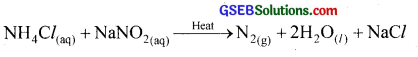(II) It is also prepared by the thermal decomposition of ammonium dichromate.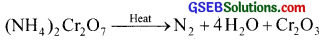This reaction is violent and accomplished by flashes of light. Therefore this reaction forms the basis of chemical volcano.

(III) Nitrogen is manufactured by the fractional distillation of pure liquid air. Air contains dinitrogen (b.p. 77K) and dioxygen (b.p. 90K). When liquid air is fractionally distilled, liquid nitrogen distills out leaving behind liquid oxygen.

Physical properties:
Nitrogen is a colourless, tasteless and odourless gas. It is slightly lighter than air. It is neither combustible nor supports combustion. It is a non-poisonous gas hut animals die in presence of nitrogen due to the deficiency of oxygen. Nitrogen atom has two stable isotopes $${ }_{7}^{14} \mathrm{~N}$$ and $${ }_{15}^{7} \mathrm{~N}$$.

Chemical properties:
At ordinary temperatures, nitrogen has very low reactivity due to the high bond dissociation energy of N ≡ N. However, at high temperatures dinitrogen combines with some metals and non-metals.

(I) Nitrogen reacts with highly electropositive metals to form nitrides even at room temperature. It reacts with less electropositive metals such as Mg, Ca, Sr etc, at red heat and with silicon at white heat to form nitrides.
6Li + N2 → 2Li3N
3Mg + N2 → Mg3N2

(II) It reacts with calcium carbide to form calcium cyanamide.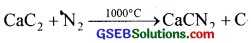(A mixture of CaCN2 and C is known as nitrolim)
Calcium cyanamide is hydrolysed in the soil to urea and ammonium carbonate. So it is used as a fertilizer.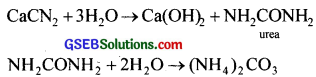(III) Nitrogen reacts with hydrogen at about 773K in presence of a spongy iron catalyst to form ammonia (Haber process).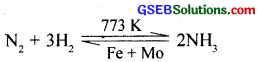(IV) Nitrogen reacts with oxygen at about 2000 K to form nitric oxide.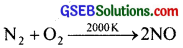Uses:

• Nitrogen is used in the manufacture of NH3, HNO3, CaCN2 and a large number of other compounds.
• It is used in providing an inert atmosphere in iron and steel industries.
• It is used for filling electric lamps.
• Liquid nitrogen is used as a refrigerant to preserve biological materials, food items and in cryosurgery.Question 6.
How is ammonia manufactured industrially?
(I) In the laboratory, ammonia is prepared by heating an ammonium salt with a strong alkali like NaOH.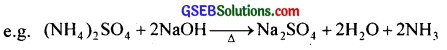NH4Cl + NaOH → NaCl + H2O + NH3

(II) Ammonia is manufactured by Haber process which involves the reaction.
N2 + 3H2 ⇄ 2NH3; ∆fH° = -46.1 kJ mol-1
This reaction is reversible, exothermic and is accompanied by a decrease in volume. Therefore, the most favourable conditions for maximum yield of ammonia according to Le Charelier’s principle are

(a) Low temperature:
The optimum temperature has been found to be nearly 773 K. (At low temperature the rate of reaction is very low.)

(b) High pressure:
The optimum pressure is nearly 200 atm.

(c) Catalyst:
Finely divided iron with molybdenum or potassium oxide as promotor. The flow chart for the production of ammonia is given below.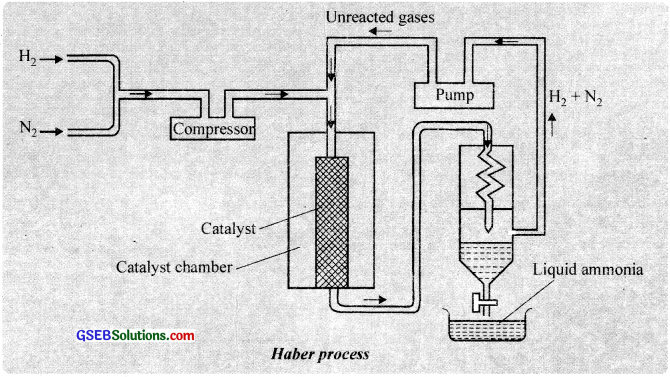Structure of Ammonia:
In ammonia, nitrogen atom undergoes sp3 hybridisation and forms four hybrid orbitais. The three half-filled hybrid orbitals overlap with 1s orbitais of H to form NH3. This NH3 molecule is trigonal pyramidal with nitrogen atom at the apex. It has three bond pairs and one lone pair as shown in the figure.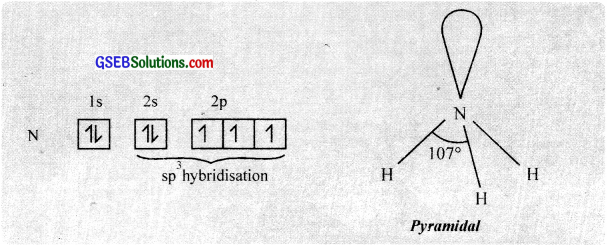Physical properties:
Ammonia is a colourless gas with a characteristic pungent smell (ammoniacal smell). It is lighter than air. Ammonia can be easily liquefied under pressure. In the solid and liquid states, ammonia is associated with intermolecular hydrogen bonding.

Chemical properties:

(I) Basic nature:
Aqueous solutions of ammonia are basic in nature and turn red litmus blue due to the formation of OH ions. Ammonia gas and aqueous ammonia react with acids to form salts.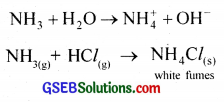(II) Formation of complex compounds:
Due to the presence of a lone pair of electrons on the nitrogen atom, ammonia behaves as a Lewis hase. Thus it forms coordinate compounds with
many metal ions.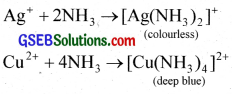(III) Aqueous ammonia precipitates metals as hydroxides. This propeiy’ is used in qualitative analysis.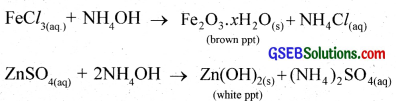Uses:
Ammonia is used

• In the manufacture of fertilizers like urea, ammonium nitrate, ammonium phosphate, ammonium sulphate etc.
• In the manufacture of HNO3, Na2CO3 etc.
• As a laboratory reagent.
• In household cleaning agents.
• Liquid ammonia is used as a refrigerant.Question 7.
Illustrate how copper metal can give different products on reaction with HNO3.
On heating copper with dil.HNO, gives nitric oxide.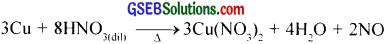On heating copper with conc.HNO3 gives nitrogen dioxide.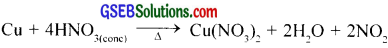Question 8.
Give the resonating structures of NO2 and N2O5?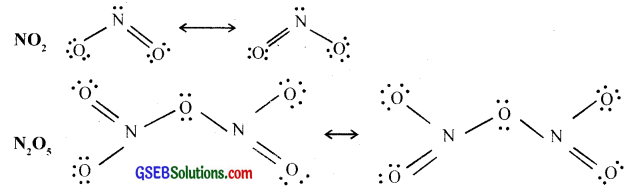Question 9.
The HNH angle value is higher than HPH, HAsH and HSbH angles. Why?
[Hint: Can be explained on the basis of sp3 hybridisation in NH3 and only s-p bonding between hydrogen and other elements of the group].
According to VSEPR theory, the lone pair-bond pair repulsion is larger than bond pair-bond pair repulsion. As a result, the tetrahedral shape is distorted and the bond angle becomes less than tetrahedral angle (109°28′). Electronegativity of N is maximum in group 15, so bond pairs of electrons lie much closer to N in NH3. The force of repulsion between the adjacent bond pairs is also high so the HNH bond angle value is higher in NH3 than HPH, HAsH and HSbH angles.

Question 10.
Why does R3P = O exist but R3N = O does not (R = alkyl group)?
Due to the absence of d orbitals, N cannot form pπ – dπ multiple bonds and hence cannot expand its covalency beyond four.

Question 11.
Explain why NH3 is basic while BiH3 is only feebly basic?
Atomic size of N(70pm) is much smaller than that of Bi (148 pm). Therefore, electron density on the N-atom is much higher than that on Bi-atom. Consequently, the tendency of N in NH3 to donate the pair of electrons is much higher than that of Bi in BiH3. Thus, NH3 is basic while BiH3 is only feebly basic.

Question 12.
Nitrogen exists as diatomic molecule and phosphorus as P4 Why?
Due to the small size of N atom, it can form pπ-pπ multiple bonds and exists as a diatomic molecule (N = N). But because of the large size of P atom, it cannot form pπ – pπ multiple bonds. It forms P – P single bonds and exists as P4 tetrahedron.Question 13.
Write main differences between the properties of white phosphorus and red phosphorus?
Elemental phosphorus is obtained by heating phosphate rock with coke and silica in an electric furnace at about 1770 K. The phosphorus thus formed is white phosphorus. The phosphorus thus obtained is stored underwater to prevent it from reaction with air.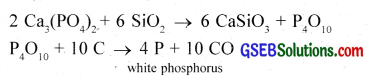Allotropic forms of phosphorus:
Phosphorus exists in three main allotropic forms- white, red and black phosphorus.

(I) White pliosplwrus (Yellow phosphorus):
It is the most common allotropic form of phosphorus. White phosphorus is formed by the condensation of phosphorus from gaseous or liquid states. It is a soft waxy solid with garlic smell and is not soluble in water hit soluble in CS2. It is highly reactive and spontaneously ignites in air and hence stored underwater.

This is because of angular strain in the P4 molecule where the angles are only 60°. The four P atoms occupy the corners of a regular tetrahedron as shown in the figure. It glows in dark and this property gives the element its name phosphorus (means light bringing (Greek)). It readily catches fire in air to give dense white fumes of P4O10.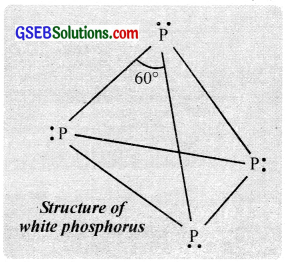P4 + 5O2 → P4O10

(II) Red phosphorus:
When white phosphorus is heated at 573 K in an inert atmosphere for several days gives, red phosphorus. Red phosphorus is a hard crystalline solid without any smell and non-poisonous. It is less reactive than white phosphorus and is insoluble in both water and CS2. It consists of tetrahedral units of P4 linked to one anotherto constitute linear chains as shown in the figure.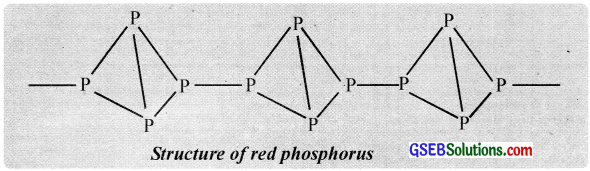(III) Black phosphorus:
It is a non-reactive form of phosphorus. α – black phosphorus is formed when red phosphorus is heated at 803K in a sealed tube. When white phosphorus is heated to 470K under high pressure of 1200 atm in an inert atmosphere gives β – black phosphorus. It lias a layer-type structure in which each layer consists of phosphorus atoms.

Question 14.
Why does nitrogen show catenation properties less than phosphorus?
Since the N – N bond is much weaker than the P – P bond, N shows catenation properties less than P.

Question 15.
Give the disproportionation reaction of H3PO3.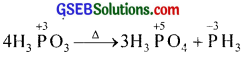Question 16.
Can PCl5 act as an oxidising as well as a reducing agent? Justify.
The oxidation state of P in PCl5 is +5 and it cannot increase its oxidation number beyond +5.
∴ PCl5 will not act as a reducing agent. But P in PCl5 can reduce its oxidation number from +5 to +3 or to some lower value. Thus it can act as an oxidising agent.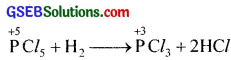Question 17.
Justify the placement of O, S, Se, Te and Po in the same group of the periodic table in terms of electronic configuration, oxidation state and hydride formation?
The elements of group 16 are collectively called chalcogens.
(i) Elements of group 16 have six valence electrons each. The general electronic configuration of these elements is ns2 np4, where n varies from 2 to 6.

(ii) Oxidation state: As these elements have six valence electrons (ns2np4), they should display an oxidation state of -2. However, only oxygen predominantly shows the oxidation state of-2 owing to its high electronegativity. It also exhibits the oxidation state of -1(H2O2), zero (O2) and +2 (OF2).
However, the stability of the -2 oxidation state decreases on moving down a group due to a decrease in the electronegativity of the elements. The heavier elements of the group show an oxidation state of +2, +4 and +6 due to the availability of d-orbitals.

(iii) Formation of hydrides: These elements from hydrides of formula H2E, where E = O, S, Se, Te, Po. Oxygen and sulphur also form hydrides of type H2E2. These hydrides are quite volatile in nature.

Question 18.
Why is dioxygen a gas but sulphur a solid?
Due to small size and high electronegativity, oxygen can form pπ – pπ multiple bonds and exists as diatomic O2 molecule. The O2 molecules are held together by weak van der Waal’s forces and is a gas at room temperature. Because of large size, sulphur does not form pπ – pπ multiple bonds and it forms only S – S single bonds. Also, sulphur has high tendency for catenation than oxygen. Thus sulphur exists as S8 molecule and are held together by strong intermolecular forces of attraction. Thus, sulphur is a solid at room temperature.Question 19.
Which aerosols deplete ozone?
Chlorofluorocarbons. e.g. From (CF2Cl2) depletes the O3 layer by supplying chlorine free radicals (Cl.) which convert O3 to O2.

Question 20.
Describe the manufacture of H2SO4 by contact process?
Theory
The process involves the following steps.
(I) Preparation of sulphur dioxideby burning sulphur.
S + O2 → SO2

(II) Oxidation of sulphur dioxide to sulphur trioxide catalytically with atmospheric oxygen. This reaction is reversible and exothermic.
2SO2 + O2 ⇄ 2SO3; ∆H = 196.6 kJ

(III) Preparation of oleum by absorbing SO3 in sulphuric acid.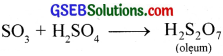It is diluted with sufficien amount of water to get sulphuric acid of required concentration.
H2S2O7 + H2O → 2H2SO4

conditions for Maximum yield:
The complete equation for the oxidation of sulphur trioxide is represented below.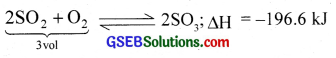According to Le Chatelier’s principle, the favourable conditions for maximum yield are

1. Since the forward reaction is exothermic. a low Iemperawre favours the oxidation of SO2 to SO3. The optimum temperature of 723 K gives better yield.
2. Since the reaction proceeds with a decrease in volume, high pressure favours the process. Better yield is obtained at 2 atm.
3. To increase the rate of reaction at low temperature, a catalyst must be used. The commonly used catalysts are platinum. ferric oxide and vanadium pentoxide (V2O5).
4. To get better yield of SO3 excess of oxygen is used. The plant used is shown in the figure.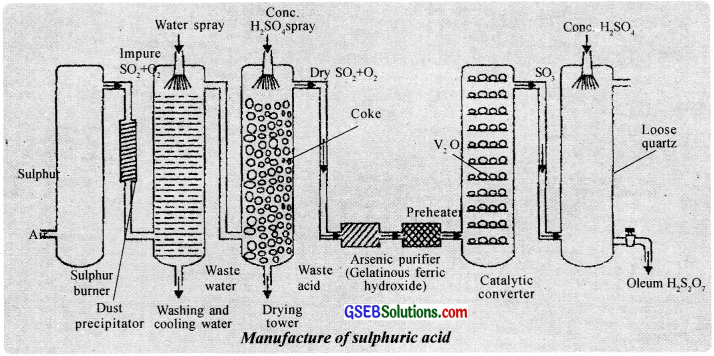Question 21.
How is SO2 an air pollutant?
SO2 dissolves in rainwater and produces acid rain. Also, the presence of SO2 in the atmosphere causes cardiac and respiratory diseases (asthma, bronchitis etc.), eye irritation etc. and damages plant cell membrane, chlorophyll etc.

Question 22.
Why are halogens strong oxidising agents?
Due to high electronegativity, low negative electron gain enthalpy and low bond dissociation enthalpy halongs have a strong tendency to accept electrons and get reduced. Thus they act as oxidising agents.

Question 23.
Explain why fluorine forms only one oxoacid, HOF?
Due to high electronegativity, small size and absence of vacant d orbitals, fluorine cannot attain oxidation states of +3, +5, +7 etc. and hence forms only HOF.Question 24.
Explain why in spite of nearly the same electronegativity, oxygen forms hydrogen bonding while chlorine does not?
Oxygen has smaller size than chlorine and hence the electron density per unit volume on oxygen atom is much higher than that on Cl atom. (Smaller size favours hydrogen bonding). Thus, oxygen forms hydrogen bonds while chlorine does not.

Question 25.
Write two uses of ClO2.
ClO2 is an oxidising agent and chlorinating agent. It is used as a bleaching agent for paper pulp and textiles and in water treatment.

Question 26.
Why are halogens coloured?
This is due to absorption of radiations in visible region which results in the excitation of outer electrons to higher energy level. By absorbing different quanta of radiation, they display different colours.

Question 27.
Write the reactions of F2 and Cl2 with water?
F2 is a strong oxidising agent and it oxidises H2O to O2 or O3.
2F2 + 2H2O → 4HF + O2
3F2 + 3H2O → 6HF + O3
While Cl2 reacts with H2O to form hydrochloric acid and hypochlorous acid.
Cl2 + H2O → HCl + HClOQuestion 28.
How can you prepare Cl2 from HCl and HCl from Cl2? Write reactions only?
Cl2 can be prepared by treating HCl with MnO2, KMnO4 or K2Cr2O7.
4HCl + MnO2 → MnCl2 + 2H2O + Cl2
HCl can be prepared by the action of H2 and Cl2 in presence of sunlight.
H2 + Cl2 → 2HCl

Question 29.
What inspired N. Bartlett for carrying out a reaction between Xe and PtF6?
The synthesis of O2PtF6 inspired Bartlett to prepare XePtF6 as Xe and oxygen have nearly the same ionisation enthalpies.

Question 30.
What are the oxidation states of phosphorus in the following?

1. H3PO3
2. PCl3
3. Ca3P2
4. Na3PO4
5. POF3

1. +3
2. +3
3. -3
4. +5
5. +5

Question 31.
Write balanced equations for the following:

1. NaCl is heated with sulphuric acid in the presence of MnO2
2. Chlorine gas is passed into a solution of Nal in water.

1. 2NaCl + MnO2 + 3H2SO4 → 2NaHSO4 + MnSO4 + 2H2O + Cl2
2. 2NaI + Cl2 → 2NaCl + I2Question 32.
How are xenon fluorides XeF2, XeF4 and XeF6 obtained?
(I) Fluorides:
Xenon fluorides are prepared by the direct reaction of xenon and fluorine under different conditions such as catalyst, heat, electric discharge and ultraviolet radiation.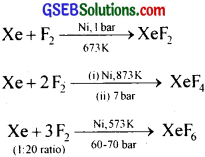Xenon fluorides react with fluoride ion acceptors like PF5, SbF5 etc. to give cationic species and fluoro anions.
XeF2 + PF5 → [XeF]+ [PF6]
XeF4 + SbF5 → [XeF3]+ [SbF6]

(II) Oxides:
Xenon trioxide is obtained by the hydrolysis of XeF4 or XeF6.
6XeF4 + 12H2O → 4Xe + 2 XeO3 + 24HF + 3O2
XeF6 + 3H2O → XeO3 + 6HF
XeO3 is a powerful explosive and oxidising agent in an aqueous solution.

(III) Oxy fluorides:
The oxy fluorides of xenon are formed by the partial hydrolysis of xenon fluorides.
XeF4 + H2O → XeOF2 + 2HF
XeF6 + H2O → XeOF4 + 2HF

Structure of xenon compounds:
The structure and molecular geometry of Xe compounds can be explained on the basis of VSEPR theory. Some examples are shown below.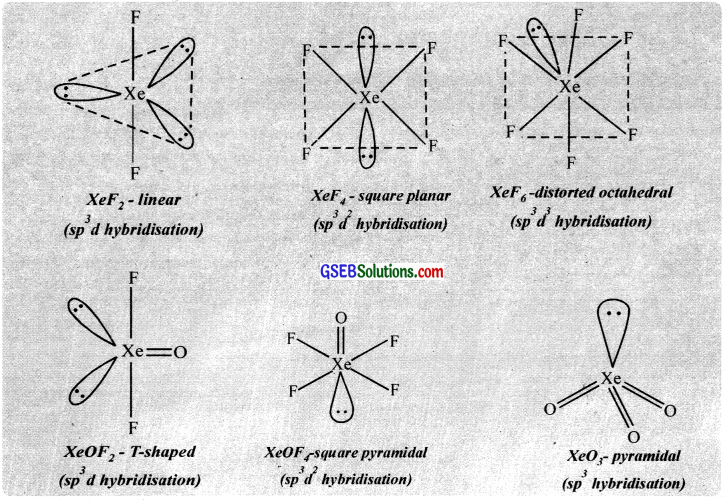Clathrates:
When certain organic or inorganic compounds are crystallised in presence of noble gases, noble gases get entrapped in the cavities of the lattice. These cage-like compounds are called clathrates. Except for helium and neon, all other elements form clathrates.

Uses of noble gases:

(I) Helium:

• Because of its lightness and non-flammability, helium is used for filling airships and observation balloons.
• It is used as a cooling medium (cryogenic agent) in gas-cooled nuclear reactors.
• It is used for providing an inert atmosphere in the welding of metals and alloys.
• It is used to produce and sustain powerful superconducting magnets which form an essential part of modem NMR spectrometers and MRI (Magnetic resonance imaging) systems for clinical diagnosis.
• It is used as diluent for oxygen in modern diving apparatus because of its low solubility in blood.

(II) NeO:

• Neon is used for filling discharge tubes for advertisements and optical decorations.
• It is used in safety devices.

(III) Argon:

• Argon is used for filling electric bulbs.
• Pure argon is used in gas chromatography.

(IV) Kr-He mixture is used in flashbulbs tòr high-speed photography.

(V) Radon is used in the treatment of cancer.

Question 33.
With what neutral molecule is ClO isoelectronic? Is that molecule a Lewis base?
ClF Since ClF can combine further with F to form ClF3, ClF is a Lewis base.Question 34.
How are XeO3 and XeOF4 prepared?
The gases Helium (He), Neon (Ne), Argon (Ar), Krypton (Kr), Xenon (Xe), and Radon (Rn) belong to group 18 of the periodic table. These are also called noble gases. Of these, Rn is a radioactive element. Each period in the periodic table ends with a noble gas. Except for helium, the atoms of all other noble gases have eight electrons in the valence shell. For helium, the outer electronic configuration is 1s2 and for other elements, it is ns2np6 (where n = 2 to 6).

Due to the inert nature of noble gases, they always occur in a free state. All the noble gases, except radon, are present in the air in small amounts. The number of noble gases present in the atmosphere (in percent by volume) is given below. He (5.2 × 10-4),Ne(1.8 × 10-3), Ar(0.93), Kr(1.4 × 10-3). Xe(8.7 × 10-6).

Helium is present to the extent of 2 to 7% in natural gas which, at present is the most important source. Radon does not occur in nature, as it. being highly radioactive decays rapidly. He, Ne, and Ar are found in the water of certain springs.

Neon, argon, krypton, and xenon are obtained as byproducts of liquefaction of air and separation of its constituents by fractional distillation. Radon is obtained b the radioactive disintegration of radium 226.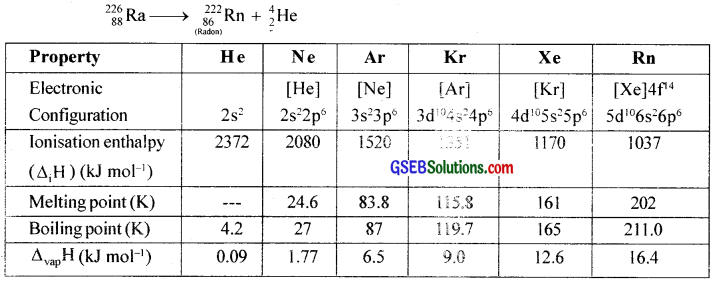Question 35.
Arrange the following in the order of property indicated for each set:

1. F2, Cl2, Br2, I2 – increasing bond dissociation enthalpy.
2. HF < HCl < HBr < HI – increasing acid strength.
3. NH3, PH3, ASH3, SbH3, BiH3 – increasing base strength.

1. I2 < F2 < Br2 < Cl2
2. HF < HCl < HBr < HI
3. BiH3 ≤ SbH3 < AsH3 < PH3 < NH3

Question 36.
Which one of the following does not exist?
1. XeOF4
2. NeF2
3. XeF2
4. XeF6
2. NeF2Question 37.
Give the formula and describe the structure of a noble gas species which is isostructural with:

1. ICl4
2. IBr2
3. BrO3

1. XeOF4
2. XeF2
3. XeO3

Question 38.
List the uses of neon and argon gases?
The gases Helium (He), Neon (Ne), Argon (Ar), Krypton (Kr), Xenon (Xe) and Radon (Rn) belong to group 18 of the periodic table. These are also called as noble gases. Of these, Rn is a radioactive element. Each period in the periodic table ends with a noble gas. Except helium, the atoms of all other noble gases have eight electrons in the valence shell. For helium, the outer electronic configuration is 1s2 and for other elements it is ns2np6 (where n = 2 to 6).

Due to the inert nature of noble gases, they always occur in free state. All the noble gases, except radon are present in air in small amounts. The number of noble gases present in the atmosphere (in percent by volume) is given below. He (5.2 × 10-4),Ne(1.8 × 10-3), Ar(0.93), Kr(1.4 × 10-3). Xe(8.7 × 10-6). Helium is present to the extent of 2 to 7% in natural gas which, at present. is the most important source. Radon does not occur in nature, as it. being highly radioactive decays rapidly. He, Ne and Ar are found in the water of certain springs.

Neon, argon, krypton and xenon are obtained as byproducts of liquefaction of air and separation of its constituents by fractional distillation. Radon is obtained b the radioactive disintegration of radium 226.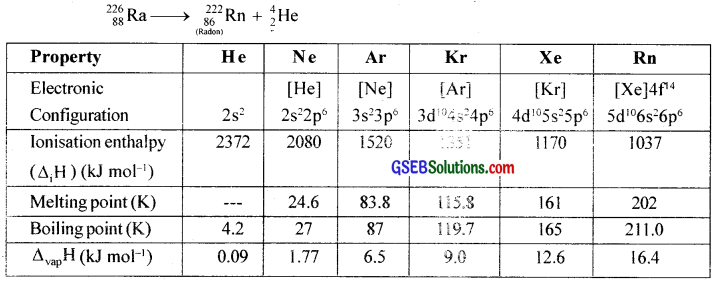### GSEB Class 12 Chemistry The p-Block Elements Additional Important Questions and Answers

Question 1.
NH3 is manufactured by passing a mixture of nitrogen and hydrogen, at a pressure of 200 atm, over a catalyst at 750 K. Under these conditions, about 15% of the gases are converted to NH3.
(a) Write an equation for the reaction and state whether it is exothermic or endothermic?
(b) Name the catalyst. Why is a catalyst used in this reaction?
(c) What happens to the gases which are not converted to ammonia?
(d) The yield of ammonia is increased if the process is carried out at
(I) even higher pressures and
(II) lower temperature. Explain why these conditions are not used in the industry.
(a)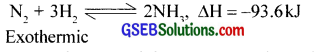(b) Spongy iron-containing Mo or K2O is used as a catalyst. A catalyst increases the rate of reaction.
(c) The unreacted N2 and H2 are sent back to the catalyst chamber.
(d) At low temperatures the rate of reaction becomes very slow.Question 2.
Match the following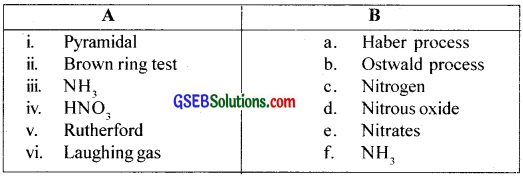(i) f
(ii) e
(iii) a
(iv) b
(v) c
(d) d

Question 3.
Nitric acid is manufactured by the catalytic oxidation of ammonia. The reactions which take place are represented by the equations (i) and (ii) below. (i) 4NH3 + 5O2 → 4NO + H2O
(ii) 4NO + 3O2 + 2H2O → 4HNO3
a. How many molecules of water would have to be formed in order to balance equation (i)?
b. Name the catalyst used in the manufacture of nitric acid.
c. Is the catalyst essential for the reaction represented by equation (i) or that represented by equation (ii), or both of these reactions?
d. Describe fully, one test for nitrate.
a. 6H2O
b. Platinum
c. Equation (i) requires catalyst.
d. Nitrates give brown ring test with Fe2+ ions in the presence of cone. H2SO4. This is based upon the tendency of Fe2+ to reduce nitrates to nitric oxide which reacts with Fe2+ to form brown coloured complex.

The test is usually performed by adding dilute FeSO4 solution to an aqueous solution containing NO3 ion and then adding conc.H2SO4 slowly along the sides of the test tube. A brown ring at the interface between the solution and sulphuric acid is formed.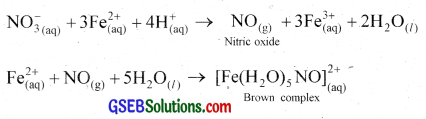Uses:

1. Nitric acid is used in the manufacture of various fertilizers (ammonium nitrate. nitrophosphates etc.), explosives (TNT nitroglycerine etc), plastics, fibres, dyestuffs etc.
2. It is used as a laboratory reagent and as an oxidising agent.
3. It is also used in the purification of silver and gold.
4. It is used as an oxidiser in rocket fuels, pickling of stainless steel and etching of metals.Question 4.
For a question, to write an example of a molecule showing sp3d hybridisation, one student wrote NCl5 as the answer.

1. Do you consider it as the correct answer? Justify.
2. Give the hybridisation of ‘P’ in PCl3 and explain the shape of the molecule.
3. Illustrate the dehydrating property of P2O5 by using H2SO4.

1. No. For sp3d hybridisation, ‘d’ orbital is necessary. Since nitrogen atom has no d orbitals, NCl5 is not an example for sp3d hybridisation.

2. In PCl5, P undergoes sp3 hybridisation. PCl3 has pyramidal structure and has one lone pair of electrons as shown in the figure.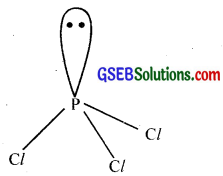3. P2O5 can liberate water molecule from a compound.
eg. 2H2SO4 + P4O100 → 2SO + 4HP03
Here H2SO4 is dehydrated.

Question 5.
Does phosphorus exist in three allotropic forms?

1. Write down the names of the allotropic forms of phosphorus,
2. Draw the structure of P4 molecule.
3. The hydride of nitrogen is known as ammonia. Write the name of the hydride of phosphorus,
4. How is it prepared?

1. The three allotropic forms of phosphorus are white phosphorus, red phosphorus and black phosphorus.
2.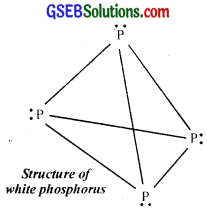3. Phosphine
4. Phosphine is prepared by heating white phosphorus with 40% solution of caustic soda in an inert atmosphere of CO2 or coal gas.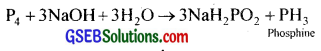Question 6.

1. Give the structure and names of any four oxyacids of phosphorus.
2. Orthophosphoric acid is a tribasic acid while phosphorus acid is a dibasic acid. Give reason.

1.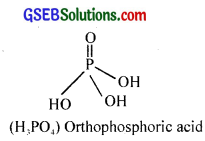2.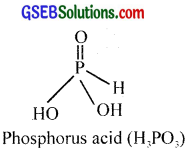3.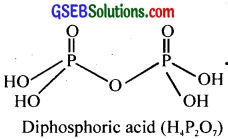4.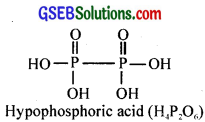Question 7.

1. Draw the structure of sulphur molecule.
2. What are the allotropic forms of sulphur?

1.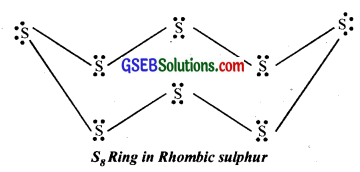2. Rhombic sulphur, monoclinic sulphur and plastic sulphur.

Question 8.
The acidic strength of hydrogen halides in aqueous solution written by three students in an examination are given below.
Appu: HF > HCl > HBr > HI
Chinnu: HI > HBr > HCl > HF
Balu: HCl > HF > HBr > HI
1. Who wrote the correct order?
1. Chinnu wrote the correct order. HI is stronger than HCl.
2. The hydrogen halides are HF, HCl, HBr and HI. In the increasing order of acidity, the hydrogen halides are HF < HCl < HBr < HI
i.e., HI is the strongest acid. As we move down from HF to HI, the size of halogen attached to hydrogen increases. Thus H-X bond length increases while bond dissociation energy decreases. So it is easy to remove hydrogen as H+ ion from HI. So acid strength increases from HF to HI.Question 9.
After discussion about the acidity of oxyacids of halogens, Ramu wrote the strength of acids in the following order.
HOCl < HClO2 < HClO3 < HClO4

1. Is this order of acid strength correct or not?
3. Draw the structure of all these oxyacids.

1. Yes
2. The oxy acids of chlorine are HClO, HClO2, HClO3 and HClO4. In the increasing order of acidity, the Oxy acids of chlorine are arranged as
HClO < HClO2 < HClO < HClO4

HClO4 is more acidic than HClO. According to the Lowry-Bronsted concept, the conjugate acid of a strong base is weak and conjugate base of a strong acid is weak. When we consider the conjugate base of oxyacids of halogen, the stability of anions are in the following order.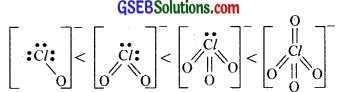ClO4 is the weakest base and ClO is the strongest base. So HClO4 is the strongest acid and HClO is the weakest acid.
3.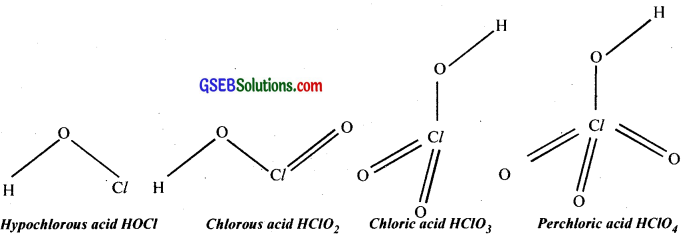Question 10.
A scientist conducted a series of experiments with xenone and fluorine. The three different conditions used are given below.
A. Ni at 400° C
B. Ni at 400° C and acetone.
C. Ni at 400° C and 200 atm.

1. Identify the products obtained in each case.
2. Write the chemical equation.
3. Draw the structure of the compounds obtained.
1. The compound obtained are XeF2, XeF4 and XeF6 respectively.
2.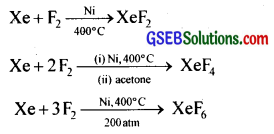3.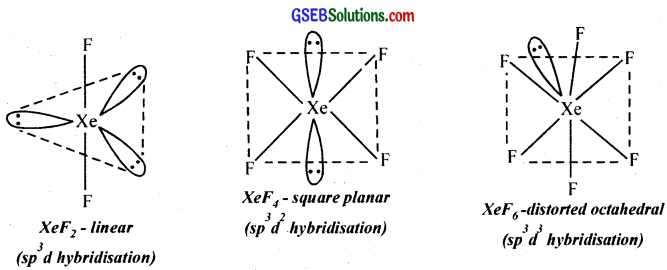Question 11.
Account for the following:

1. Molecular nitrogen is not particularly reactive.
2. Water has a higher boiling point than H2S.
3. Nitrogen forms no pentahalide, unlike phosphorus.
4. Cl2O7 is formed but no such fluorine compound is formed.

1. In a nitrogen molecule, two nitrogen atoms are held together by a triple bond and the energy required to break the triple bond is very high (984 kJ). Therefore N2 is chemically inert.
2. Due to intermolecular hydrogen bonding in water, it has a high boiling point compared to H2S.
3. Phosphorus, because of the availability of vacant ‘d’ orbital can extend the oxidation state to +5 and forms PCl5. While nitrogen due to the unavailability of vacant ‘d’ orbital cannot extend its covalency.
4. Fluorine can’t increase its oxidation number upto +7 because of the non-availability of electrons for bond formation.Question 12.

1. Draw the structure of H2SO4
2. Write a chemical reaction which shows the dehydrating nature of H2SO4.
3. Why is H2SO4 called the king of chemicals?

1.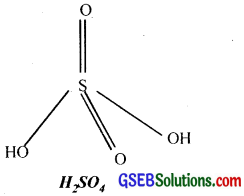2. Glucose gets charred when treated with cone. H2SO4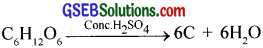3. H2SO4 is called the king of chemicals because of its extensive applications in various manufacturing processes like the manufacture of fertilizers, various reagents, explosives, and its uses in petroleum refining, metallurgy, storage batteries, etc.

Question 13.
a. What are interhalogen compounds?
b. Give the method of preparation and structure of

• ClF3
• IF7
• ClF5